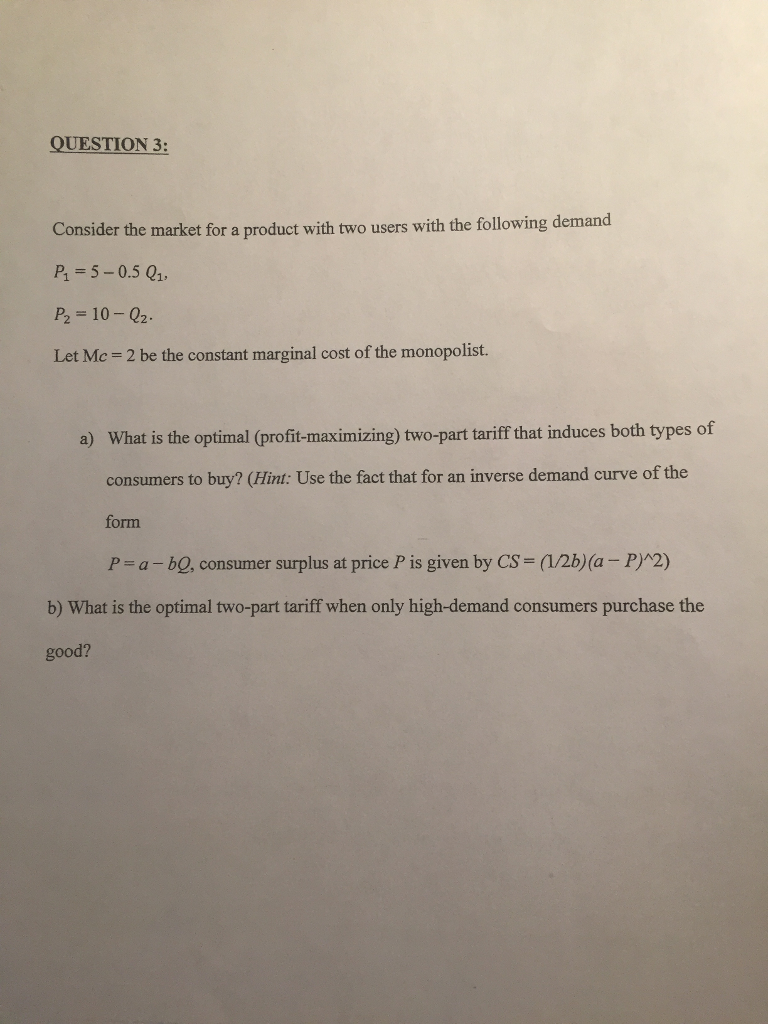### Create an Account

Home / Questions / Consider the market for a product with two users with the following demand P_1 = 5-0.5 Q_1...

# Consider the market for a product with two users with the following demand P_1 = 5-0.5 Q_1, P_2=10-Q_2. Let Mc = 2 be the constant marginal cost of the monopolist. What is the optimal (profit

Consider the market for a product with two users with the following demand P_1 = 5-0.5 Q_1, P_2=10-Q_2. Let Mc = 2 be the constant marginal cost of the monopolist. What is the optimal (profit-maximizing) two-part tariff that induces both types of consumers to buy? What is the optimal two-part tariff when only high-demand consumers purchase the good?Jul 12 2021 View more View LessSubscribe To Get Solution